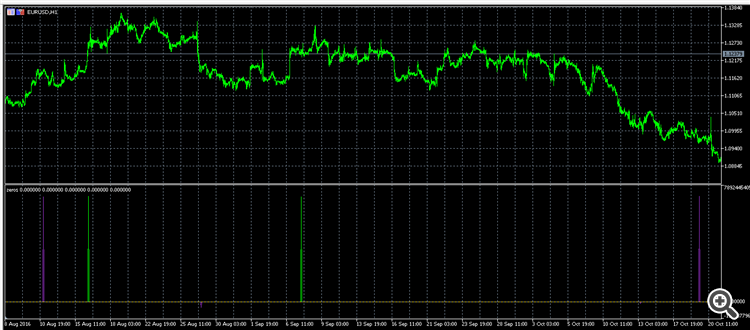Indicators' PLOT_EMPTY_VALUE not working properly2627

I have encountered some strange behavior with initiating an indicator. Setting the PLOT_EMPTY_VALUE to 0 does not seem to work properly, with values other than 0 appearing in the data window.

I have made the following indicator to test different empty value setting ways:

```#property description "not zeros at all"
#property version "1.0"

#property indicator_separate_window
#property indicator_buffers 5
#property indicator_plots   5

//buffers
double Buffer0[],Buffer1[],Buffer2[],Buffer3[],Buffer4[];

int OnInit()
{
//0
SetIndexBuffer(0,Buffer0,INDICATOR_DATA);
PlotIndexSetDouble(0,PLOT_EMPTY_VALUE,0);
PlotIndexSetInteger(0,PLOT_DRAW_TYPE,DRAW_LINE);
PlotIndexSetInteger(0,PLOT_LINE_COLOR,clrTomato);
PlotIndexSetInteger(0,PLOT_DRAW_BEGIN,1);
PlotIndexSetString(0,PLOT_LABEL,"0");

//(double)0
SetIndexBuffer(1,Buffer1,INDICATOR_DATA);
PlotIndexSetDouble(1,PLOT_EMPTY_VALUE,(double)0);
PlotIndexSetInteger(1,PLOT_DRAW_TYPE,DRAW_LINE);
PlotIndexSetInteger(1,PLOT_LINE_COLOR,clrLime);
PlotIndexSetInteger(1, PLOT_SHOW_DATA, true);
PlotIndexSetInteger(1,PLOT_DRAW_BEGIN,1);
PlotIndexSetString(1,PLOT_LABEL,"(double)0");

//0.0
SetIndexBuffer(2,Buffer2,INDICATOR_DATA);
PlotIndexSetDouble(2,PLOT_EMPTY_VALUE,0.0);
PlotIndexSetInteger(2,PLOT_DRAW_TYPE,DRAW_LINE);
PlotIndexSetInteger(2,PLOT_LINE_COLOR,clrDarkOrchid);
PlotIndexSetInteger(2,PLOT_DRAW_BEGIN,1);
PlotIndexSetString(2,PLOT_LABEL,"0.0");

//EMPTY_VALUE
SetIndexBuffer(3,Buffer3,INDICATOR_DATA);
PlotIndexSetDouble(3,PLOT_EMPTY_VALUE,EMPTY_VALUE);
PlotIndexSetInteger(3,PLOT_DRAW_TYPE,DRAW_LINE);
PlotIndexSetInteger(3,PLOT_LINE_COLOR,clrGold);
PlotIndexSetInteger(3,PLOT_DRAW_BEGIN,1);
PlotIndexSetString(3,PLOT_LABEL,"EMPTY_VALUE");

//zero
const double zero = 0.0;
SetIndexBuffer(4,Buffer4,INDICATOR_DATA);
PlotIndexSetDouble(4,PLOT_EMPTY_VALUE,zero);
PlotIndexSetInteger(4,PLOT_DRAW_TYPE,DRAW_LINE);
PlotIndexSetInteger(4,PLOT_LINE_COLOR,clrLightGray);
PlotIndexSetInteger(4,PLOT_DRAW_BEGIN,1);
PlotIndexSetString(4,PLOT_LABEL,"zero");

IndicatorSetString(INDICATOR_SHORTNAME, "zeros");
IndicatorSetInteger(INDICATOR_DIGITS, Digits()+1);

return(INIT_SUCCEEDED);
}

int OnCalculate(const int rates_total,
const int prev_calculated,
const datetime &time[],
const double &open[],
const double &high[],
const double &low[],
const double &close[],
const long &tick_volume[],
const long &volume[],
{
return rates_total;
}```

And the plot I get is this:The spikes are indicative of the indicator lines not zeroing out. The spikes can be different between consecutive compilations of the indicator(without changing anything in the code).

The only way that seems to work is the last one with the use of a double variable.

Is this a bug or is there any other way to properly set the empty value for an indicator buffer?38403

Bug in your code. You NEED to initialize the buffers.2627

Alain Verleyen:
Bug in your code. You NEED to initialize the buffers.

Do I do this with the int  ArrayInitialize( double  array[],double  value); method?

And if yes at which point? After the SetIndexBuffer call in OnInit()?38403

Dimitrios Vezeris:

Do I do this with the int  ArrayInitialize(double  array[],double  value); method?

Yes.

And if yes at which point? After the SetIndexBuffer call in OnInit()?

No. In OnCalculate(), when prev_calculated=0